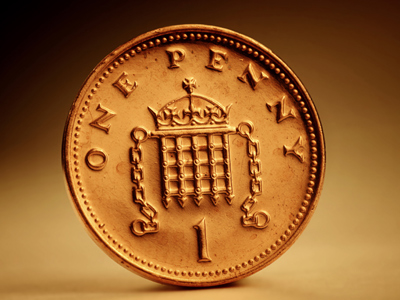One penny = £0.01, that's one-hundredth of a pound.

# Decimal Numbers 3 (Easy)

Welcome to the third in our Easy section of Eleven Plus maths quizzes on decimal numbers. In it we look in particular at the value of those digits placed after the decimal point. You know that, in whole numbers, the columns read right to left are for ones, tens, hundreds, thousands etc. Well. In decimal numbers, it’s almost the same, but with no ‘ones’ column - and in reverse!

Perhaps this will help make it clearer:

• First column after decimal point = tenths
• Second column after decimal point = hundredths
• Third column after decimal point = thousandths
• Fourth column after decimal point = ten-thousandths

The fractions get smaller and smaller as you read left to right.

Have a go at this quiz and see how well you understand decimals. Once you get all questions right first time, why not move on to our next quiz for more of a challenge!

1.
What is the value of the 5 in the number 891.256?
5 thousandths
5 hundredths
5 tenths
50 tenths
Five hundredths are the same as one-twentieth
2.
What is three-thirds represented as a decimal number?
0.999
1
3.333
0.666
Three-thirds make one!
If all the numbers after the decimal point are zeros, then you can leave them out
3.
Which digit in the number below is the same as 10 thousandths?
98,765.312
The one
The nine
The two
The eight
10 thousandths are the same as one-hundredth, and would be shown in decimal as 0.01
4.
What are the place positions of the numbers in the decimal parts of the numbers given?
3,101.028
8 is in the tenths place position, 2 is in the hundredths place position, 0 is in the thousandths place position
2 is in the tenths place position, 8 is in the hundredths place position, 0 is in the thousandths place position
8 is in the tenths place position, 0 is in the hundredths place position, 2 is in the thousandths place position
0 is in the tenths place position, 2 is in the hundredths place position, 8 is in the thousandths place position
Decimal numbers are in columns just like ordinary numbers - but backwards! Thousands, hundreds, tens, ones, tenths, hundredths, thousandths
5.
What is one-third represented as a decimal number?
0.3
0.4
0.25
0.333
One-third as a decimal is 1 ÷ 3 = 0.333… the threes are recurring, which means they go on forever!
6.
What is the value of the 2 in the number 101.942?
2 thousandths
2 hundredths
2 tenths
2 ten-thousandths
The third column after the decimal point represents thousandths, not hundredths
7.
What is two-thirds represented as a decimal number?
0.666
0.6
0.667
0.66
One-third as a decimal is 0.333... so two-thirds is 0.333... + 0.333... = 0.666... which we round up to 0.667
8.
What are the place positions of the numbers in the decimal parts of the numbers given?
27.396
6 is in the tenths place position, 9 is in the hundredths place position, 3 is in the thousandths place position
3 is in the tenths place position, 6 is in the hundredths place position, 9 is in the thousandths place position
3 is in the tenths place position, 9 is in the hundredths place position, 6 is in the thousandths place position
9 is in the tenths place position, 6 is in the hundredths place position, 3 is in the thousandths place position
TIP:
The first number to the right of the dp is in the tenths place position, e.g. 3 in 7.3
The second number to the right of the dp is in the hundredths place position, e.g. 9 in 7.39
The third number to the right of the dp is in the thousandths place position, e.g. 6 in 7.396
9.
What are the place positions of the numbers in the decimal parts of the numbers given?
106.275
2 is in the tenths place position, 7 is in the hundredths place position, 5 is in the thousandths place position
7 is in the tenths place position, 5 is in the hundredths place position, 2 is in the thousandths place position
5 is in the tenths place position, 7 is in the hundredths place position, 2 is in the thousandths place position
2 is in the tenths place position, 5 is in the hundredths place position, 7 is in the thousandths place position
There is no 'ones' column after the decimal point - it's tenths first, then hundredths, thousandths etc.
10.
What is the value of the 8 in the number 0.872?
8 thousandths
8 hundredths
8 tenths
8 ones
TIP:
The first number to the right of the dp is in the tenths place position, e.g. 8 in 0.8
The second number to the right of the dp is in the hundredths place position, e.g. 7 in 0.87
The third number to the right of the dp is in the thousandths place position, e.g. 2 in 0.872
Author:  Frank Evans